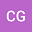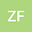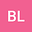An Elastic-Electrical Joint Method for Fracture Evaluation in Anisotropic Formation
•••• +1Zhenzhen Fan
Chang'an University
Author Profile## Abstract

The formation evaluation of fractured shale has been a challenging topic in unconventional exploration engineering due to the complicated formation conditions with strong anisotropy. The inversion result for the fracture and the water/oil saturation could be less reliable if only the single-physics model of either elastic or electrical is considered. In this work, an elastic-electrical joint method involving both elastic modulus and resistivity tensor is proposed to characterize the fractures as well as predict the water saturation for the anisotropic formation. In this method, we employ the three-order tensor invariants to characterize the orthogonal resistivity tensor matrix of anisotropic model. Both the elastic modulus and the electrical tensors of each fractured model are computed with a FEM based multi-physics simulator. By varying the fracture shape, alignment and the porosity (or water saturation), the cross relation between elastic modulus and electrical tensor invariants in terms of fracture shape is established. Therefore, the water saturation can be predicted using a tensor-revised Archie’s law which was proposed in our previous work. We validate the proposed joint method using numerical experiments conducted on a set of idealized fracture geometries (e.g. needle-like fractures and disk-like fractures). The joint curve of elastic and electrical tensor invariants is able to predict the fractures shape accurately with a known porosity. In addition, the tensorial electrical parameters of formation can be inversed from the joint curve as the elastic tensor and the electrical scalar is determined by measurement. It provides an alternate mean to obtain the electric tensor (e.g. resistivity tensor) when the anisotropic measurement is not available in some practical engineering scenarios. This work indicate that the proposed elastic-electrical joint method can provide significant theoretical and engineering value for well logging interpretation as well as formation evaluation.